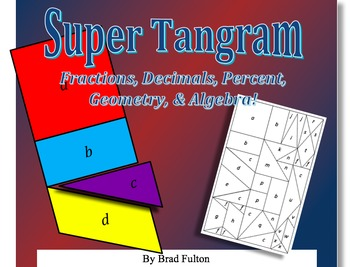# Super Tangram: Fractions, Decimals, Percent, Geometry, and AlgebraSubject
Resource Type
File Type

PDF

(7 MB|16 pages)
Standards
• Product Description
• StandardsNEW
This is a wonderful follow-up activity to the “Tangram Math” lessons offered on my TeachersPayTeachers store. However, it can also be used as an engaging stand-alone exploration. Students will learn the properties and vocabulary of polygons as well as integrate the learning of fraction, decimal, and percent equivalencies. As they work, they will incorporate algebraic reasoning and discourse in a seamless process.
There are three activities that use the super tangram, and you and your students may think of even more applications. They are
a) assigning values with part/whole representations (fraction, decimal, percent)
b) finding areas and/or perimeters of polygons
c) writing algebraic equations
Vocabulary: acute, area, congruent, equilateral, flip, isosceles, obtuse, parallelogram, rectangle, rhombus, right angle, rotate, scalene, similar, square, trapezoid, triangle
Represent proportional relationships by equations. For example, if total cost 𝘵 is proportional to the number 𝘯 of items purchased at a constant price 𝘱, the relationship between the total cost and the number of items can be expressed as 𝘵 = 𝘱𝘯.
Recognize and represent proportional relationships between quantities.
Use ratio reasoning to convert measurement units; manipulate and transform units appropriately when multiplying or dividing quantities.
Find a percent of a quantity as a rate per 100 (e.g., 30% of a quantity means 30/100 times the quantity); solve problems involving finding the whole, given a part and the percent.
Use ratio and rate reasoning to solve real-world and mathematical problems, e.g., by reasoning about tables of equivalent ratios, tape diagrams, double number line diagrams, or equations.
Total Pages
16 pages
Included
Teaching Duration
1 Week
Report this Resource to TpT
Reported resources will be reviewed by our team. Report this resource to let us know if this resource violates TpT’s content guidelines.